# A New Introduction to Modal Logic erratum

Just noticed a significant and potentially confusion causing error regarding multiply modal logics in this nonetheless excellent text. On pages 217-218 in my 1996 edition Hughes and Cresswell write:

For instance we might have a necessity operator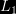$L_{1}$, say, which is stronger than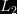$L_{2}$ in the sense that$L_{1} p \supset L_{2}p$. The canonical model for such a system would obey the restriction that for all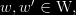$w, w' \in \text{W},$ if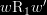$w\text{R}_{1}w'$ then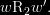$w\text{R}_{2}w'.$

Now, that should be for all$w, w' \in \text{W},$ if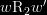$w\text{R}_{2}w'$ then$w\text{R}_{1}w'$.